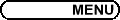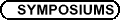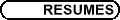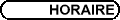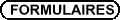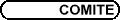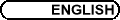Contributed Papers Session / Communications libres
(Org: WRS Sutherland, Dalhousie University)

CLAUDIA DANIELA CALIN, University of Alberta, Edmonton, Alberta T6G 2G1
Coagulation equation with unbounded kernels, particle sources and sinks

During the past few years, increasing attention and effort have been given to the mathematical theory of coagulation equations which model the formation of large particles by the coalesence of smaller particles. Coagulation equations arise in a number of problems in the physical and polymer sciences, colloid chemistry, aerosol physics. One interesting aspect of the coagulation equation, that occurs for certain coagulation kernels, is that mass need not be conserved for all time. The phenomenon whereby conservation of mass breaks down in finite time is known as gelation and is physically interpreted as being caused by the appearance of an infinite "gel" or "superparticle".

MEGAN DEWAR, Carleton University, Ottawa, ON K1S 1S2
Universal Cycles and Block Designs

The term Universal Cycle was introduced by Chung, Diaconis and Graham in 1992. A Universal Cycle (Ucycle) is defined as follows: Let Fn be a family of combinatorial objects of rank k with m = |Fn|. Let A be a fixed alphabet from which the elements of each F Î Fn are selected. A Ucycle for Fn is a cycle (x0, ..., xm-1), where xi Î A (for i=0,...,m-1) and where every element of Fn appears exactly once as some k-block of this cycle. The most well-known type of Ucycle is for the n-bit binary numbers-this is a De Bruijn cycle of order n. In this talk we discuss what is known about the existence and construction of Ucycles for various combinatorial objects. We begin with a look at the published research which focusses on Ucycles for k-subsets of n-sets and k-permutations of n-sets. We then look at Ucycles for block designs-a family of combinatorial objects previously not considered.

ZHIPENG DUAN, Memorial University of Newfoundland, P. O. Box 40, Faculty of Engineering, St. John's, NL A1B 3X5
Impingement Air-Cooled Plate Fin Heat Sinks-Pressure Drop Model

The performance of impingement air-cooled plate fin heat sinks differs significantly from that of parallel flow plate fin heat sinks. A semi-empirical zonal model has been published to predict impingement flow in plate fin heat sinks. This model is not convenient to apply since it involves a large number of equations. A simple impingement flow pressure drop model based on developing laminar flow in rectangular channels is proposed. To test the validity of the model, experimental measurements of pressure drop are performed with heat sinks of various impingement inlet widths, fin spacings, fin heights and airflow velocities. It was found that the predictions agree with experimental data within 20 numbers less than 1200. The simple model is suitable for parametric design studies.

JEANETTE EDGE, Memorial University of Newfoundland
Fully Developed Flow of Non-Newtonian Fluids in Non Circular Ducts

There has been much research conducted on the behavior of Newtonian fluids in the process industry for pipes of various cross sectional shapes. Shear stress related correlations have been derived for the elliptical, rectangular, polygonal, and annulus shaped ducts. It has been shown that for a given value of aspect ratio the dimensionless values of shear stress for each shape combination collapse on to one another to a single value. Furthermore, for the case of Newtonian fluid flow, effects of non-dimensionalization techniques using various length scales have been analyzed. It has been determined that through the use of the square root of cross sectional area for the characteristic length, as opposed the hydraulic diameter, shear stress values indicated an increase in accuracy while minimizing the effect of varying duct shapes.

Despite the research that has been completed for Newtonian fluids, little attention has been made on modeling Non-Newtonian fluids in similar situations. Some researchers have devoted time and effort in the analysis of Non-Newtonian fluids and their reaction to independent cross sectional shapes. There has not, however, been an analysis performed in which the various geometric shapes are compared in their non-dimensional form.

Most text books commonly include tabulated and/or graphical data describing the most common shapes, thus there is a desire in industry to have a more robust model that can predict system characteristics as simply as possible.

The goal of conducting this research is to condense the data that has been obtained from a number of sources into a single equation that can be used to describe non-Newtonian flow for a variety of cross sectional areas. This equation will be used for all shapes where the only term that is altered is the geometric coefficient relating the shape of interest to that of fundamental geometries.

CRISTIAN ENACHE, Département de mathématiques et de statistique, Cité universitaire Québec (Québec), G1K 7P4, Canada
Some maximum principles for a class of elliptic boundary problems

The subject of this talk will be about a general class of ellipic boundary value problem on convex bounded domain with a sufficiently smooth boundary. For a functional combination of the solution and its gradient we will construct an elliptic inequality for which we can apply the Hopf maximum principles to derive a priori bounds for some quantities related to our solution without any explicit knowledge of the solution itself. We will also give some applications for geometrical or physical problems.

KRISTI HOLLOWAY, Memorial University, St. John's, NL
Numerical simulations of viscous fingering involving a single fluid

We present results of numerical simulations of the flow of a single fluid with a temperature dependent viscosity in the gap of a radial Hele-Shaw cell. The simulations are performed using the CFD software, Fluent. The cell, consisting of two parallel plates separated by a small gap, is modelled using a grid of 364520 wedge cells. The walls of the cell are maintained at a constant cold temperature and the cell is initially filled with cold glycerin. Hot glycerine enters the cell at a constant rate through a hole in the bottom plate of the cell. A fingering instability can occur via which the `hot' glycerine forms fingers that penetrate into the cold glycerine. We present results for the temperature and velocity profiles of the flow and a stability diagram which shows the initial parameters for which the flow is unstable. We also compare our numerical results with experiment.

RANIS IBRAGIMOV, Department of Applied Mathematics, University of Waterloo, Waterloo, ON N2L 3G1, Canada
Generation of Internal Tides by an Oscillating Background Flow Along a Corrugated Slope

The process of internal wave generation by the interaction of an oscillatory background flow ( U0 cos(w0 t), V0sin(w0 t),

W0 sin(w0 t) ) over three-dimensional bottom topography is investigated. The topography considered is a uniform slope with a superimposed corrugation running directly up and down the slope. Here we present analytical and numerical analysis of internal tide generation in a stratified, rotating fluid of infinite depth to better understand the energetics of the wave generation process. In our model, energy is radiated away from the bottom as internal gravity waves. Attention is primarily directed to estimating the flux of energy into the internal wave field. Since waves are generated not only at the fundamental frequency w0, but also at all of its harmonics, the energy flux for both low and high frequency waves is considered. It is shown that the acoustic limit approximation (the limiting case in which the tidal excursion, U0/w0, is much less than the scale of the topography) gives a reasonable approximation for the behaviour of the energy as the function of the slope, inertial frequency and the basic flow. The nature of water parcel paths over the corrugations is also considered.

BOUALEM KHOUIDER, University of Victoria, Math. and Stat., 3800 Finnerty Road (Ring Road) Victoria, BC V8P 5C2, Canada
A numerical model for the barotropic-baroclinic interactions of equatorial waves

We present a high resolution numerical model for the barotropic-baroclinic interactions of the equatorial waves in a channel which essentially preserves energy and geostrophic steady states. A non-linear incompressible 2d flow and a linear sallow water system are obtained through Galerkin projection of the non-linear beta-plane primitive equations on the barotropic and the first baroclinic modes. The two systems exchange energy through non trivial interaction terms though total energy is conserved. A high resolution conservative scheme which preserves geostrophic steady states is used for each piece: the quasi-steady wave propagation algorithm of R. LeVeque for the baroclinic mode and the central incompressible scheme of Levy-Tadmor for the barotropic mode. The interaction terms are gathered in a single second order accurate scheme to minimize energy leakage. Validation tests and barotropic-baroclinic wave interaction runs will be presented.

Joint work with A. Majda.

GREG LEWIS, University of Ontario Institute of Technology, 2000 Simcoe Street North, Oshawa, Ontario L1H 7K4
Bifurcations in a differentially heated rotating spherical shell

We study the steady axisymmetric bifurcations that occur in a model that uses the Navier-Stokes equations in the Boussinesq approximation to describe the fluid flow in a differentially heated rotating spherical shell with radial gravity (i.e., a simple model for large-scale atmospheric dynamics).

The solutions and the corresponding eigenvalues are approximated numerically from the large sparse systems that result from the discretization of the partial differential model equations.

COLIN MACDONALD, Simon Fraser University, Burnaby, BC
Constructing High-Order Runge-Kutta Methods with Embedded Strong-Stability-Preserving Pairs

This talk will deal with the construction of fifth-order Runge-Kutta schemes with embedded third-order strong-stability-preserving (SSP) Runge-Kutta pairs. The original motivation for such pairs was to evolve Weighted Essentially Non-Oscillatory (WENO) spatial discretizations of hyperbolic conservation laws. The third-order SSP scheme would be used near shocks or discontinuities where the SSP property is useful for minimizing spurious oscillations. Also, WENO discretizations provide at most third-order in space near such non-smooth features. The fifth-order scheme would then be used in smoother regions where WENO provides fifth-order in space and SSP properties are not necessary.

I will concentrate on the specific techniques used for the construction of these Runge-Kutta pairs. I will comment briefly on the effectiveness of these embedded pairs for evolving hyperbolic conservation laws, however, these techniques are quite general and could easily be applied in other areas of study. Thus, I will finish by noting some other possible applications of these schemes and the techniques used in their construction.

FRANKLIN MENDIVIL, Acadia University, Wolfville, NS B4P 2R6
Chaos Games for Wavelet Analysis and Wavelet Synthesis

We start by discussing the classical "Chaos Game" for IFS fractals and a modification to render self-similar L2 functions. We then review the modification of this chaos game to produce an approximation of a wavelet. By suitably mixing chaos games for different translations and dilations of the mother wavelet, we can construct a chaos game to generate approximations to arbitrary L2 functions. This process can also be reversed, yielding a chaos game for generating the wavelet expansion coefficients of an L2 function.

SUSAN MOLLOY, Memorial University
Uncertainty Analysis of Powering Prediction Methods of Podded Propulsor Models

Podded propulsion is a recent innovation in ship powering. The rudder and propeller configuration raises new challenges in the extrapolation of model powering data to full-scale values. Traditional testing and prediction methods have proven unsatisfactory at capturing the complexity of the interaction between the propeller and pod. Using a series of model pods, a set of full-scale data and uncertainty analysis the traditional methods are explored and an alternative approach to analysing model tests is proposed. A full uncertainty analysis of the measuring system is included. Using Monte Carlo Simulation the relative stability of the traditional powering extrapolation method is compared to the proposed method. The sensitivity of each method to the powering parameters and correction factors is determined. Full-scale data is used to evaluate the results and an acceptable uncertainty range is proposed.

BENJAMIN ONG, Simon Fraser University
A Moving Mesh Method Based On Level Set Ideas

It has been well documented that mesh adaptation is of critical importance in the numerical solution of partial differential equations (PDEs). The accuracy and efficiency improvements often warrant the extra work entailed in implementing an adaptive mesh.

A promising direction in the field of adaptivity are the so-called moving mesh methods. In such methods, a mesh equation is solved (often simultaneously with the original differential equation) for node velocities, which move/keep the nodes concentrated in regions of rapid variation of the solution.

In practice, however, most moving mesh methods suffer from an effect known as "mesh crossing". These typically arise because of some difference approximations when calculating the mesh velocities.

I will discuss a new moving mesh method based on Level Set ideas. Such methods eliminate mesh crossings entirely. They also allow for topology change in the solution or domain. I will then show some numerical solutions of the Porous Medium Equation (PME) and Fisher's Equation using this new moving mesh method.

MATIUR RAHMAN, Department of Engineering Mathematics, Dalhousie University, Halifax, Nova Scotia B3J 2X4, Canada
Seismic response of earth dams: a theoretical investigation

In order to analyze the safety and stability of an earth dam during an earthquake, we need to know the response of the dam to earthquake ground motion so that the inertia forces that will be generated in the dam by earthquake can be derived. Once the inertia forces are known, the safety and the stability of the structure can be determined. In this paper we have investigated an analytical solution of the seismic response of dams in one dimension. In real life situation the seismic problem is three dimensional. However, for an idealized situation, we can approximate the problem to study in one dimension. The result obtained in this paper should be treated as a bench mark solution.

MATEJA SAJNA, University of Ottawa, 585 King Edward, Ottawa, ON
Almost self-complementary graphs

A graph X of even order is said to be almost self-complementary if it is isomorphic to the graph obtained from the complement of X by removing the edges of a 1-factor. The study of almost self-complementary graphs was first suggested by Brian Alspach, who proposed the determination of all possible orders of almost self-complementary circulant graphs. A paper by Dobson and Sajna, where this problem is solved for a particularly "nice" subclass of almost self-complementary circulants (called cyclically almost self-complementary) shows that the structure of almost self-complementary graphs is much more complex than that of self-complementary graphs.

In this talk, we present some basic results on almost self-complementary graphs and define three binary operations on graphs that can be used to construct infinite families of almost self-complementary graphs with certain desired properties. One of these constructions, for example, shows that regular almost self-complementary graphs exist for all even orders-in contrast with regular self-complementary graphs, which exist only for orders congruent to 1 modulo 4.

This is joint work with Primoz Potocnik.

SAMUEL SHEN, University of Alberta, Edmonton
The Mathematics in the IPCC Report on Detection, Prediction and Uncertainty of Climate Changes

This talk will explain some mathematics behind the science in the 2001 Report of the Intergovernmental Panel on Climate Change (IPCC) in the areas of detection, prediction, and uncertainties of climate change. Detection is to discern the signals driven by external forces, such as volcanic aerosols, from the observed climate data. Prediction is the use of numerical models in forecasting the climate conditions over a period of several decades with annual or monthly resolution. The uncertainties exist in observed data, data analysis methods, model parameters, and statistical inference, and consequently in conclusions regarding global and regional climate changes. The talk will describe the IPCC's methods and results of detection, optimal analysis of observed data, and predictions. But questions remain:

• Has detection taken into account the nonlinear interaction among the effects of greenhouse gases, volcanic aerosols, and solar irradiance?

• Have the optimization techniques taken non-stationarity into account?

• Do we have a satisfactory assessment of the errors of the observed data over the globe?

## References


C. K. Folland, N. A. Rayner, S. J. Brown, T. M. Smith, S. S. P. Shen, D. E. Parker, I. Macadam, P. D. Jones, R. Jones and N. Nicholls, Global temperature change and its uncertainties since 1861. Geophys. Res. Lett. 28(2001), 2621-2624.


IPCC Report. Cambridge University Press, 2001, Chs. 2, 9, 10 and 12.


C. E. Forest, P. E. Stone, A. P. Sokolov, M. R. Allen and M. D. Webster, Quantifying uncertainties in climate system properties with the use of recent climate observations. Science 295(2002), 113-117.


S. S. P. Shen, T. M. Smith, C. F. Ropelewski and R. E. Livezey, An optimal regional averaging method with error estimates and a test using tropical Pacific SST data. J. Climate 11(1998), 2340-2350.

MARINA V. TVALAVADZE, Department of Mathematics and Statistics, Memorial University of Newfoundland, St. John's, NL, Canada
Classification of simple decompositions of Jordan algebras and superalgebras

This research is dedicated to the classification of simple decompositions of Jordan algebras and superalgebras. By simple decomposition we understand representation of an (super)algebra as the vector sum of two proper simple subalgebras. Based on the explicit classification of simple Jordan algebras and superalgebras, it was shown that simple Jordan algebras of type H(Fn) and superalgebras of types osp(n,m), P(n), Q(n), K3 and Dt have no simple decompositions. Conversely, all simple decompositions of (super)algebras of all other types were found in the explicit matrix form.

TEYMURAZ TVALAVADZE, Department of Mathematics and Statistics, Memorial University of Newfoundland, St. John's, NL, Canada
Simple decompositions of Lie superalgebras

This talk will report on the recent classification of simple decompositions of Lie superalgebras. The similar problem is well-studied in the case of Lie algebras. Generally speaking, we consider the representation of the given simple Lie superalgebra as the sum of two proper simple subalgebras. In this research the classification of simple Lie algebras and superalgebras play a key role. In particular, it was shown that classification of simple complex Lie algebras can be extended to the case of algebraically closed field of zero characteristic. Also, all simple decompositions of classical Lie superalgebras were explicitly classified and represented in the matrix form.

NAVEEN VAIDYA, York University, 4700 Keele St., Toronto, ON M3J 1P3
Grown-in Defects Modeling of InSb Crystals

In this talk, we will present a model for grown-in point defects inside indium antimonide crystals grown by the Czochralski technique. This model includes the Fickian diffusion and recombination mechanism. This type of model is used for the first time to analyse grown-in point defects in indium antimonide crystals.

The temperature solution and the advance of the melt-crystal interface are based on a recently derived perturbation model. We study the effect of thermal flux on the point defect patterns during and at the end of the growth process. Our results show that the concentration of excessive point defects is positively correlated to the heat flux in the system. Based on the result of our two-dimensional model, we can conclude that for growing less-defect crystals of larger radius one has to reduce the heat transfer coefficient by a suitable amount from the lateral surface of the crystal.

MICHAEL WAITE, McGill University, Department of Atmospheric and Oceanic Sciences, 805 Sherbrooke St. W., Montreal, QC H3A 2K6
Numerical simulations of vortical and wave motion in stably stratified turbulence

Turbulence in a stratified fluid can be decomposed into a potential vorticity-carrying (vortical) component and an internal wave component. In the limit of strong stratification, internal waves become weakly nonlinear while vortical motion remains fully nonlinear; the two components have very different limiting dynamics.

In this talk, we contrast stratified turbulence dominated by vortical and wave motion. Numerical simulations are presented of a Boussinesq fluid, in which large-scale vortical and wave motion are forced separately over a wide range of stratifications. With vortical forcing and strong stratification, energy spectra are found to be quite different from observations in the atmosphere and ocean.

However, we argue that these results are consistent with the limiting dynamics, which for vortical motion predict decoupled layers of two-dimensional turbulence. We show that vertical decoupling breaks down at a scale of U/N where U is the RMS velocity and N is the Brunt-Väisälä frequency, in agreement with theory. These findings are contrasted with the wave-forced simulations.

STEVEN QIANG WANG, School of Mathematics and Statistics, Carleton University, Ottawa, Ontario K1S 5B6, Canada
Generalized Inflations and Null Extensions

An inflation of an algebra is formed by adding a set of new elements to each element in the original or base algebra, with the stipulation that in forming products each new element behaves exactly like the element in the base algebra to which it is attached. Clarke and Monzo have defined the generalized inflation of a semigroup, in which a set of new elements is again added to each base element, but where the new elements are allowed to act like different elements of the base, depending on the context in which they are used. Such generalized inflations of semigroups are closely related to both inflations and null extensions. Clarke and Monzo proved that, for a semigroup base algebra which is a union of groups, any semigroup null extension must be a generalized inflation, so that the concepts of null extension and generalized inflation coincide in the case of unions of groups. As a consequence, the collection of all associative generalized inflations formed from algebras in a variety of unions of groups also forms a variety.

In this paper we define the concept of a generalized inflation for any type of algebra. In particular, we allow for generalized inflations of semigroups which are no longer semigroups themselves. After some general results about such generalized inflations, we characterize for several varieties of bands which null extensions of algebras in the variety are generalized inflations, and which of these are associative. These characterizations are used to produce examples which answer, in our more general setting, several of the open questions posed by Clarke and Monzo.

This is a joint work with Shelly L. Wismath.

RONG WANG, Dalhousie University, Halifax, NS
A numerical study of time integration schemes for the cubic Schrödinger equation

The method-of-lines (MOLs) is a popular approach for the computational treatment of partial differential equations. We describe two software packages which use collocation with a B-spline basis for the spatial discretization, and which use, for the resulting differential algebraic equations (DAEs), either DASSL or RADAU5. The former is based on a family of backward differentiation formulas (BDFs), while the latter uses a fifth-order A-stable implicit Runge-Kutta scheme. These MOL packages use a sophisticated algorithm for spatial adaptation and spatial error control. We apply the software to the nonlinear cubic Schrödinger equation in one space variable. Because this problem has all the eigenvalues on the imaginary axis, A-stablity is necessary for the time integration schemes. Only first and second order BDF methods are A-stable. Computational results show that while DASSL is unable to obtain a correct solution unless we restrict the maximum order of the method to 2, RADAU5 handles the problem without difficulty.

JIN YUE, Dalhousie University
The 1856 Cayley Lemma Revisited

Arthur Cayley (1821-1895), an eminent English mathematician of the 19th century, made many significant contributions to a number of areas of mathematics, including algebra, invariant theory, projective geometry and group theory. Among his most remarkable works may be mentioned the "ten memoirs on quantics", commenced in 1854 and completed in 1878.

We will review one of the works in this series, namely "A second memoir on quantics" (Phil. Trans. Roy. Soc. London 146(1856), 101-126) that contains a result known in classical invariant theory as "Cayley's Lemma". It concerns the action of SL(2) on the space of homogeneous polynomials of degree n. We will then formulate and prove an analogue of Cayley's Lemma in the invariant theory of Killing tensors of pseudo-Riemannian geometry.